# Resources tagged with: Equivalent fractions, decimals and percentages

Filter by: Content type:
Age range:
Challenge level:

### There are 11 results

Broad Topics > Fractions, Decimals, Percentages, Ratio and Proportion > Equivalent fractions, decimals and percentages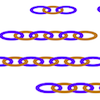##### Age 7 to 11 Challenge Level:

Can you find ways to make twenty-link chains from these smaller chains? This gives opportunities for different approaches.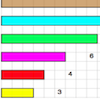### Fraction Lengths

##### Age 7 to 11 Challenge Level:

Can you find combinations of strips of paper which equal the length of the black strip? If the length of the black is 1, how could you write the sum of the strips?### Terminating or Not

##### Age 11 to 14 Challenge Level:

Is there a quick way to work out whether a fraction terminates or recurs when you write it as a decimal?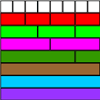### Fractional Wall

##### Age 7 to 11 Challenge Level:

Using the picture of the fraction wall, can you find equivalent fractions?### Almost One

##### Age 11 to 14 Challenge Level:

Choose some fractions and add them together. Can you get close to 1?### The Greedy Algorithm

##### Age 11 to 14 Challenge Level:

The Egyptians expressed all fractions as the sum of different unit fractions. The Greedy Algorithm might provide us with an efficient way of doing this.### Matching Fractions, Decimals and Percentages

##### Age 7 to 14 Challenge Level:

Can you match pairs of fractions, decimals and percentages, and beat your previous scores?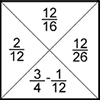### Fractions Jigsaw

##### Age 11 to 14 Challenge Level:

A jigsaw where pieces only go together if the fractions are equivalent.### Round and Round and Round

##### Age 11 to 14 Challenge Level:

Where will the point stop after it has turned through 30 000 degrees? I took out my calculator and typed 30 000 ÷ 360. How did this help?### Sept 03

##### Age 11 to 14 Challenge Level:

What is the last digit of the number 1 / 5^903 ?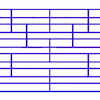### Tumbling Down

##### Age 7 to 11 Challenge Level:

Watch this animation. What do you see? Can you explain why this happens?